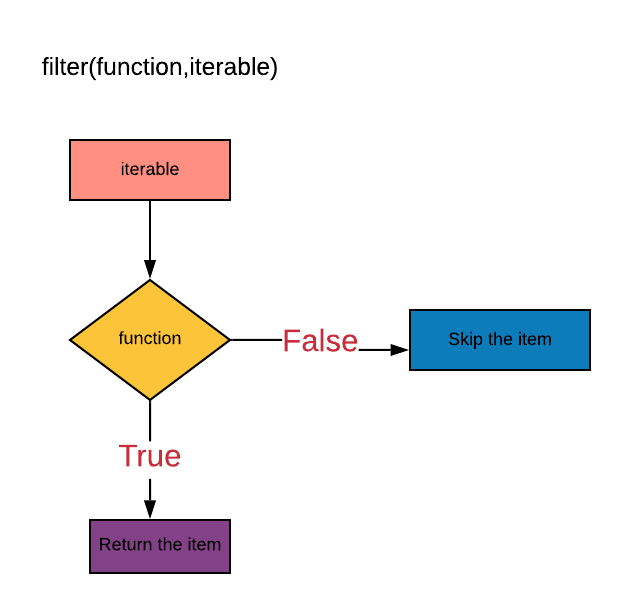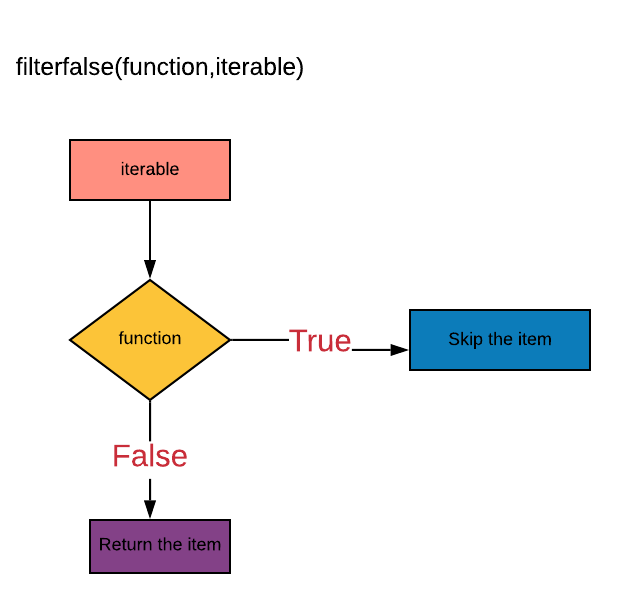# Python filter and filterfalse functions guide

## python filter function

python filter function
``````filter(function, iterable)
``````
• iterable — can be sequences like list, tuple, or container or an iterator
• function - function applied to iterable
• return type - is a filter object which is an iterator

We can access the filter object which is an iterator using below mentioned ways:

• We can convert the filter object to sequence objects list using `list()` constructor and tuple using `tuple()` constructor
• We can iterate through the filter object using python for loop
• We can access the element in the filter object using the `next()` function alsopython filter function

#### Example 1: Using user-defined function in filter()

``````def even(x):
if x % 2 == 0:
return True

numbers       = [1, 2, 3, 4, 5, 6, 7, 8, 9, 10]
filter_object = filter(even, numbers)

print(filter_object)
# output:
# <filter object at 0x7f3c713634a8>

print(type(filter_object))
# output:<class 'filter'>

list(filter_object)
# output:
# [2, 4, 6, 8, 10]
``````
• list numbers is given as an iterable.
• function even() is defined to find the even numbers.
• `filter()` will return a filter object which is an iterator
• converting the filter object to list using `list()` constructor

#### Example 2: Using lambda function in filter()

Filtering values greater than 10 from the dictionary:

``````my_dict = {'item1': 12, 'item2': 40, 'item3': 2}

filter_object = filter(lambda x: x > 10, my_dict.values())

list(filter_object)
# output:
# [12, 40]
``````
``````my_list = [5, 9, 10, 15, 25, 50]

filter_object = filter(lambda x: x > 10, my_list)

list(filter_object)
# output:
# [15, 25, 50]
``````

#### Example 3: Using None in filter()

``````my_list = [2, 5, 8, "", {}, (), [], False, None, "abc"]

filter_object = filter(None, my_list)
list(filter_object)
# output:
# [2, 5, 8, 'abc']
``````

#### Example 4: Iterating through filter object using for loop

``````my_list = ["blue", "brown", "red", "green", "back"]

filter_object = filter(lambda x: x.startswith("b"), my_list)

for item in filter_object:
print(item)

# output:
# blue
# brown
# back
``````

#### Example 5: Iterating through filter object using next() function

• we can access the next element in an iterator using the `next()` function
``````my_list = ["blue", "brown", "red", "green", "back"]

filter_object = filter(lambda x: x.startswith("b"), my_list)

print(next(filter_object))
print(next(filter_object))
print(next(filter_object))
# output:
# blue
# brown
# back

print(next(filter_object))
# output
# Traceback (most recent call last):
#   File "<stdin>", line 1, in <module>
# StopIteration
``````

## python itertools.filterfalse

python filterfalse function
``````itertools.filterfalse(predicate, iterable)
``````python filterfalse function

#### Example 1: Using lambda function in filterfalse()

``````import itertools

# iterator will filter the elements from the iterable which returns False for the given function
numbers = [1, 2, 3, 4, 5, 6, 7, 8, 9, 10]

filterfalse_object = itertools.filterfalse(lambda x: x > 4, numbers)

print(filterfalse_object)
# output:
# <itertools.filterfalse object at 0x7f3c713635f8>

list(filterfalse_object)
# output:
# [1, 2, 3, 4]
``````
• import itertools module
• define the lambda function to filter the elements greater than 4
• pass lambda function and iterable list numbers to `itertools.filterfalse()` function
• `filterfalse()` will return the elements less than 4. It will filter the elements from the iterable which returns False for the given function.
• return type is an iterator. converting to list using `list()` constructor

#### Example 2: Using user-defined function in filterfalse()

``````import itertools

def even(x):
if x % 2 == 0:
return x

numbers = [1, 2, 3, 4, 5, 6, 7, 8, 9, 10]
filterfalse_object = itertools.filterfalse(even, numbers)

list(filterfalse_object)
# output:
# [1, 3, 5, 7, 9]
``````

#### Example 3: Using None in filterfalse()

``````import itertools

# if predicate is None, returns the items that are False.
filterfalse_object = itertools.filterfalse(None, [0, 1, 2, 3, [], {}, ()])

list(filterfalse_object)
# output:
# [0, [], {}, ()]
``````